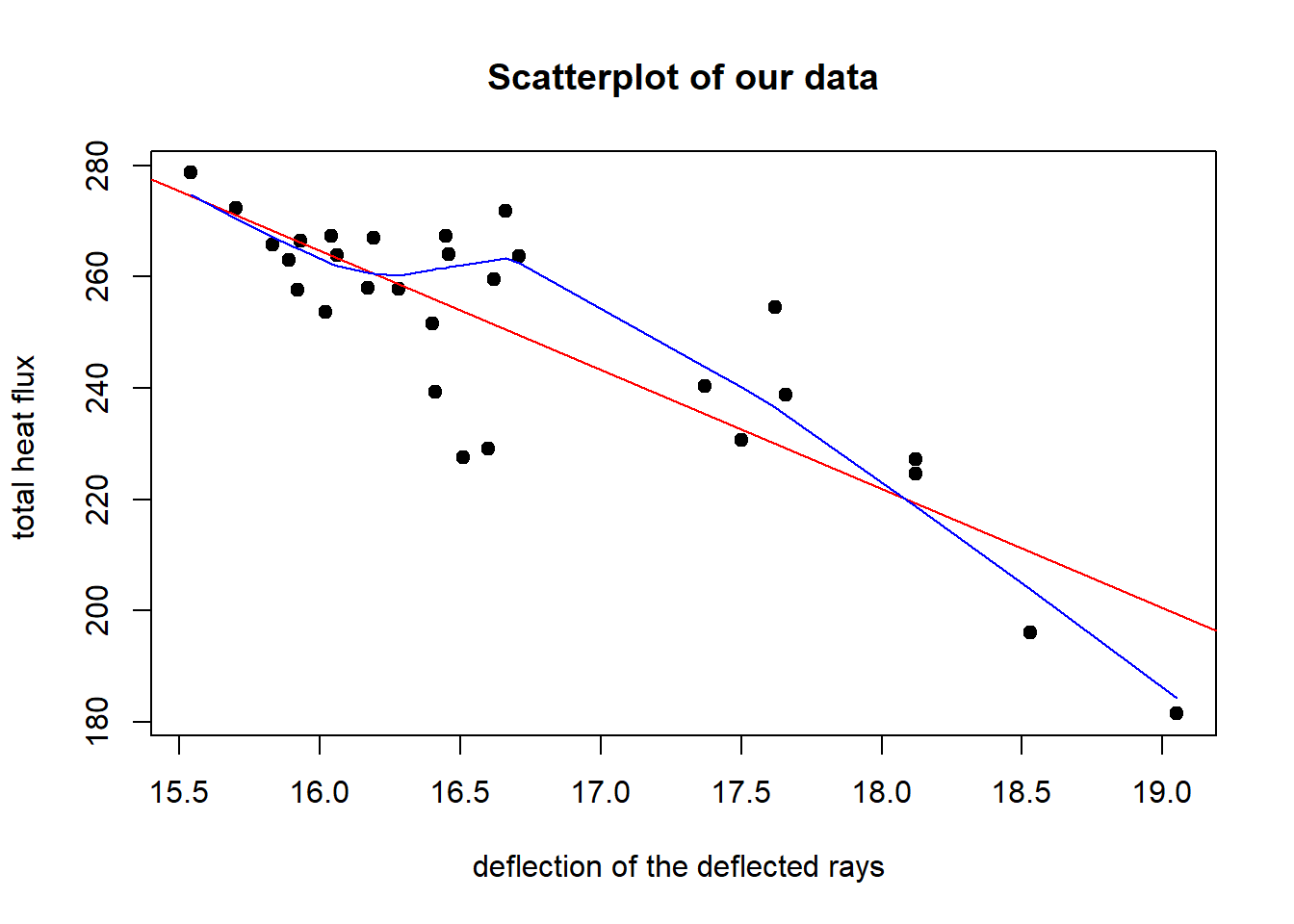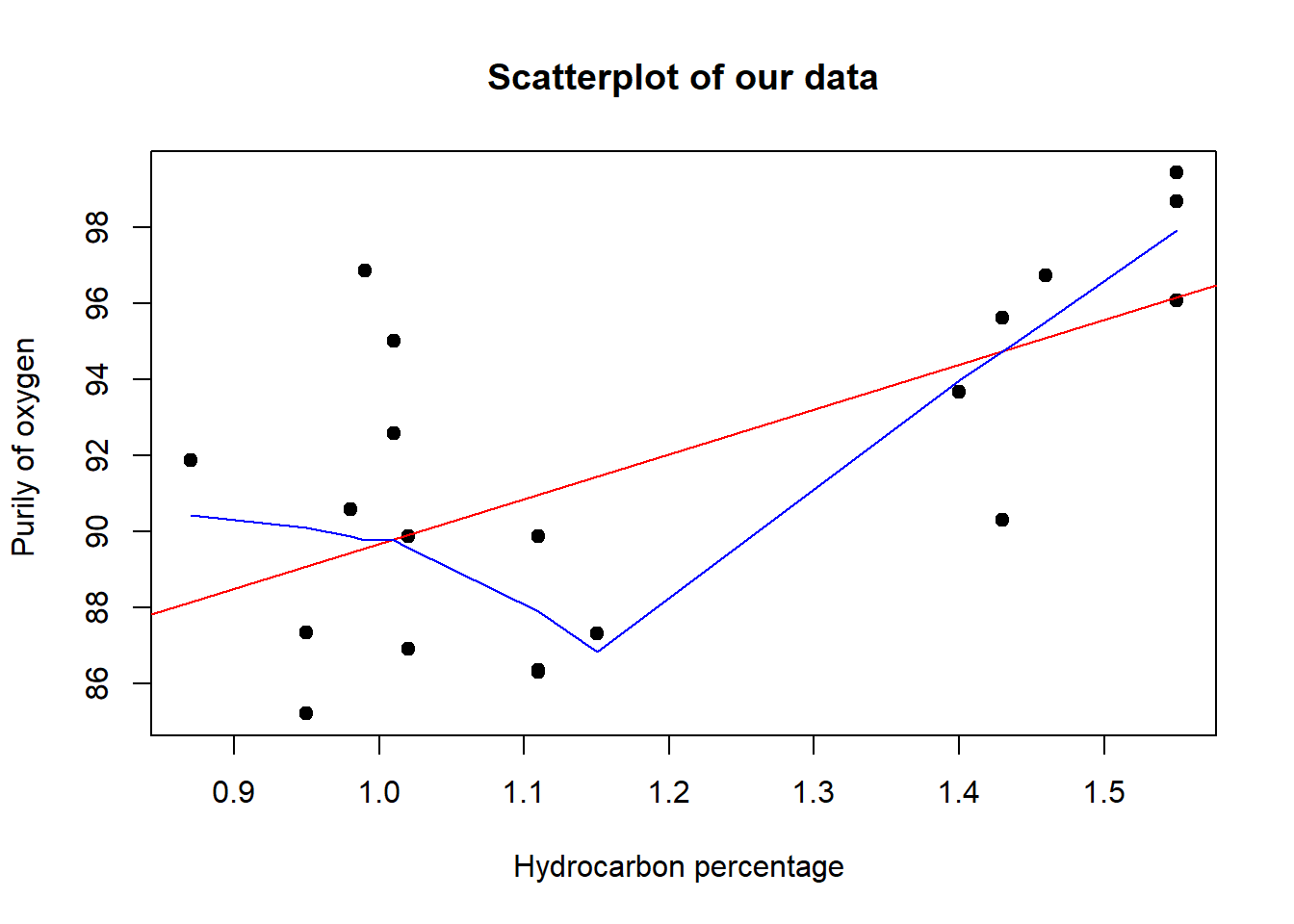## Introduction

This is my solution to some problems in chapter 2 of the book INTRODUCTION TO LINEAR REGRESSION ANALYSIS 5th edition , MONTGOMERY,PECK,VINING.

## 2.3

the first thing I should do is to plug in our data. we have y and x. y is total heat flux (measured in kilowatts) while x is radial deflection of the deflected rays (measured in milliradians )

y=c(271.8, 264, 238.8, 230.7, 251.6, 257.9, 263.9, 266.5, 229.1,
239.3, 258, 257.6, 267.3, 267, 259.6, 240.4, 227.2, 196, 278.7,
272.3, 267.4, 254.5, 224.7, 181.5, 227.5, 253.6, 263, 265.8, 263.8)

x=c(16.66, 16.46, 17.66, 17.5, 16.4, 16.28, 16.06,
15.93, 16.6, 16.41, 16.17, 15.92, 16.04, 16.19, 16.62, 17.37, 18.12,
18.53, 15.54, 15.7, 16.45, 17.62, 18.12, 19.05, 16.51, 16.02, 15.89, 15.83, 16.71)

Also I want to plot the y vs x and see the scattering plot, for better understand the data. I also fitted the plot using our SLM.

plot(x, y, main="Scatterplot of our data",
xlab="deflection of the deflected rays ", ylab="total heat flux ", pch=19)
abline(lm(y~x), col="red") # regression line (y~x)
lines(lowess(x,y), col="blue") # lowess line (x,y)### a: Fit a simple linear regression model relating total heat flux y (kilowatts) to the radial deflection of the defl ected rays x4 (milliradians).

we want to fit simple linear regression model for our data. this is simply done in R using “lm” function

model = lm(y~x)

now we want to show our model results

model
##
## Call:
## lm(formula = y ~ x)
##
## Coefficients:
## (Intercept)            x
##       607.1        -21.4

Intercept = 607.1 Slope = -21.4 so for our simple regression model, we get the equation for b y = 607.1 - 21.4x

### b: Construct the analysis-of-variance table and test for significance of regression.

to test significance of regression, we should use ANOVA test . this can be simply done using anova function in R

anova(model)
## Analysis of Variance Table
##
## Response: y
##           Df  Sum Sq Mean Sq F value    Pr(>F)
## x          1 10578.7   10579  69.609 5.935e-09 ***
## Residuals 27  4103.2     152
## ---
## Signif. codes:  0 '***' 0.001 '**' 0.01 '*' 0.05 '.' 0.1 ' ' 1

we have “Pr(>F)” of 5.935e-09 which is our p-value to compare. is less than our significance of 0.05. so we can conclude that our model is significant.

### c: Find a 99% CI on the slope

to test that variablitiy, we first need to show our model values and then do some manipulation as the following

let us first get summary of our model parameters and store it in some variable “m”

m= summary(model)

print this variable will show us its content now

m
##
## Call:
## lm(formula = y ~ x)
##
## Residuals:
##      Min       1Q   Median       3Q      Max
## -26.2487  -4.5029   0.5202   7.9093  24.5080
##
## Coefficients:
##             Estimate Std. Error t value Pr(>|t|)
## (Intercept)  607.103     42.906  14.150 5.24e-14 ***
## x            -21.402      2.565  -8.343 5.94e-09 ***
## ---
## Signif. codes:  0 '***' 0.001 '**' 0.01 '*' 0.05 '.' 0.1 ' ' 1
##
## Residual standard error: 12.33 on 27 degrees of freedom
## Multiple R-squared:  0.7205, Adjusted R-squared:  0.7102
## F-statistic: 69.61 on 1 and 27 DF,  p-value: 5.935e-09

if we need to get 99% confidence interval for the slope we do it as following

c(m$coefficients[2,1] - qt(0.995, length(x)-2) * m$coefficients[2,2], m$coefficients[2,1] + qt(0.995, length(x)-2) * m$coefficients[2,2])
##  -28.50995 -14.29497

or we can use confint function in R

confint(model, 'x', level=0.99)
##       0.5 %    99.5 %
## x -28.50995 -14.29497

so we see that our confience interval is (-28.50995, -14.29497)

### d: Calculate R^2

. it is easy to calcuate the R^2 value , it is given in model summary that our “Multiple R-squared” is 0.7205 so our R^2 = 0.7205

### e: Find a 95% CI on the mean heat fl ux when the radial defl ection is 16.5 milliradians

we want to find a 95% CI on the mean heat flux when the radial deflection is 16.5 milliradians. we can do this using our model prediction. use predict function in R to give us this thing.

predict(model, data.frame(x=16.5), interval="confidence",level=0.95)
##        fit      lwr      upr
## 1 253.9627 249.1468 258.7787

so our confidence interval when radial deflection is 16.5 milliradians is (249.1468, 258.7787)

## 2.7

the first thing I should do is to plug in our data. we have y and x. y is Purily of oxygen while x is Hydrocarbon percentage.

y2=c(86.91,89.85,90.28,86.34,92.58,87.33,86.29,91.86,95.61,89.86,96.73,99.42,98.66,
96.07,93.65,87.31,95,96.85,85.2,90.56)
x2=c(1.02,1.11,1.43,1.11,1.01,0.95,1.11,0.87,1.43,1.02,1.46,1.55,1.55,1.55,1.40,
1.15,1.01,0.99,0.95,0.98)

Also I want to plot the y vs x and see the scattering plot, for better understand the data. I also fitted the plot using our SLM.

plot(x2, y2, main="Scatterplot of our data",
xlab="Hydrocarbon percentage ", ylab="Purily of oxygen ", pch=19)
abline(lm(y2~x2), col="red") # regression line (y~x)
lines(lowess(x2,y2), col="blue") # lowess line (x,y)### a: Fit a simple linear regression model to the data

we repeat samething like problem 2.3 to fit the simple linear regression model

model2=lm(y2 ~ x2)

now let us explore what our model

model2
##
## Call:
## lm(formula = y2 ~ x2)
##
## Coefficients:
## (Intercept)           x2
##       77.86        11.80

Intercept = 77.86

Slope = 11.80

so for our simple regression model, we get the equation for b y = 77.86 + 11.80x

### b: Test the hypothesis H0 : ??1 = 0.

now I want to test the null hypothesis that beta_1 = 0

we get model summary to get our p-value to do this test

summary(model2)
##
## Call:
## lm(formula = y2 ~ x2)
##
## Residuals:
##     Min      1Q  Median      3Q     Max
## -4.6724 -3.2113 -0.0626  2.5783  7.3037
##
## Coefficients:
##             Estimate Std. Error t value Pr(>|t|)
## (Intercept)   77.863      4.199  18.544 3.54e-13 ***
## x2            11.801      3.485   3.386  0.00329 **
## ---
## Signif. codes:  0 '***' 0.001 '**' 0.01 '*' 0.05 '.' 0.1 ' ' 1
##
## Residual standard error: 3.597 on 18 degrees of freedom
## Multiple R-squared:  0.3891, Adjusted R-squared:  0.3552
## F-statistic: 11.47 on 1 and 18 DF,  p-value: 0.003291

p-value = Pr(>|t|) = 3.54e-13 which is less that 0.05 so we can reject null hypothesis.

### c: Calculate R2

from the model summary in part b we can see that Multiple R-squared = 0.3891 so our R^2 = 0.3891

### d: Find a 95% CI on the slope.

I repeat the samething I did in problem 2.3

s = summary(model2)

to get CI interval we use confint function in R

confint(model2, 'x2', level=0.95)
##       2.5 %   97.5 %
## x2 4.479066 19.12299

so our CI interval is (4.479066,19.12299)

### e: Find a 95% CI on the mean purity when the hydrocarbon percentage is 1.00.

we use predict function in R to get our model prediction like part e in 2.3

predict(model2, data.frame(x2=1), interval="confidence",level=0.95)
##        fit      lwr      upr
## 1 89.66431 87.51017 91.81845

so our CI interval is (87.51017,91.81845)